2 de Jun de 2023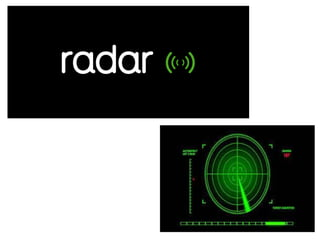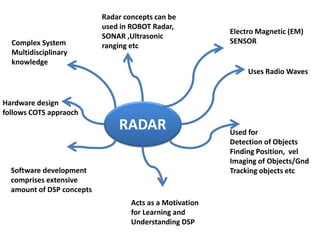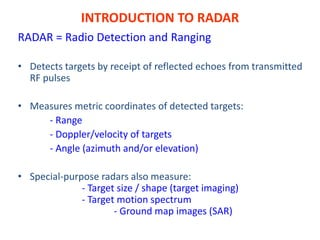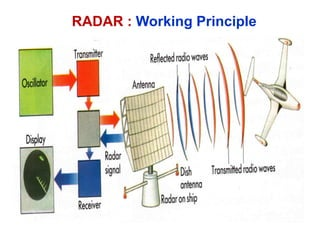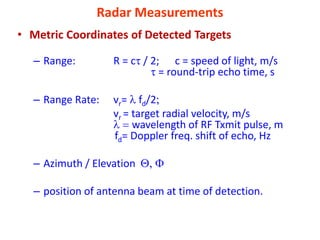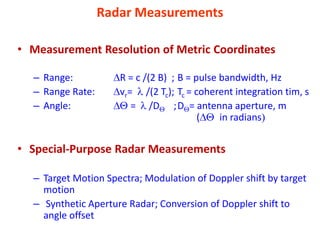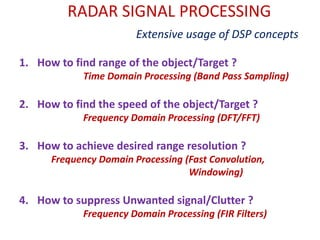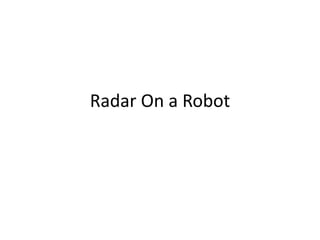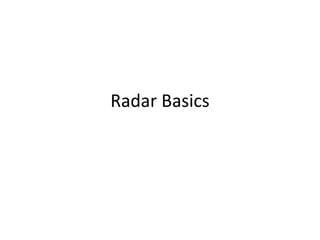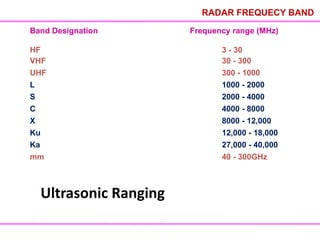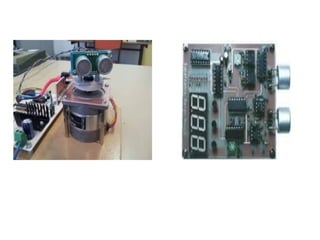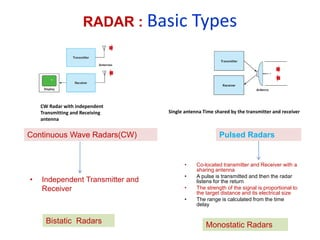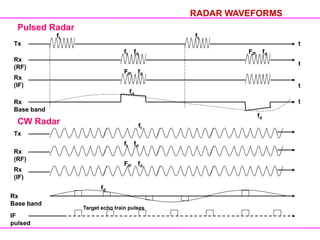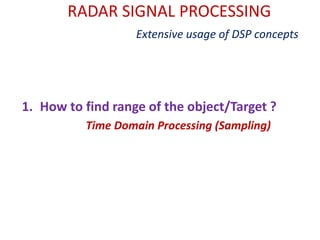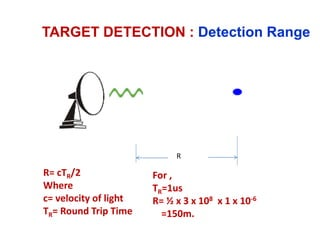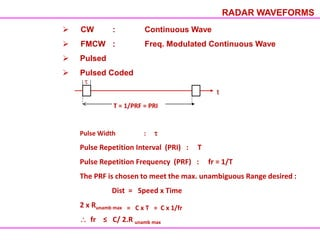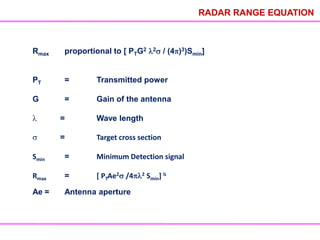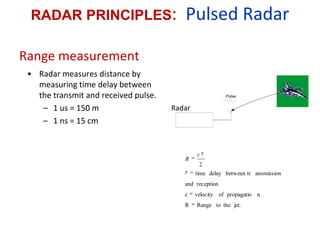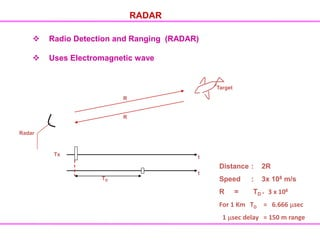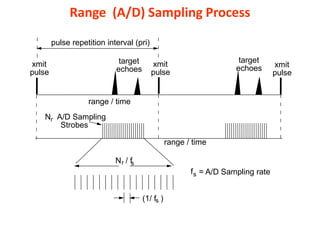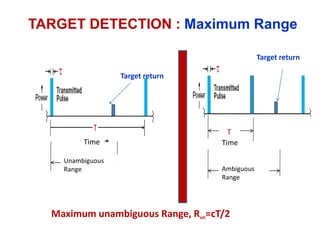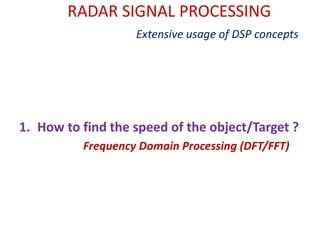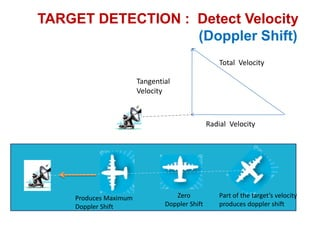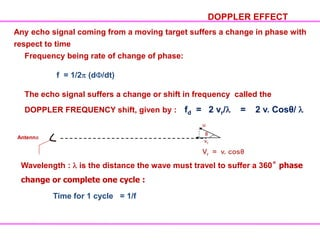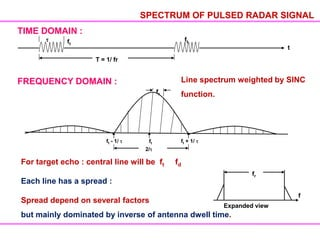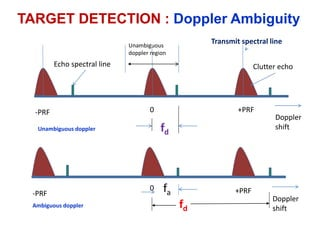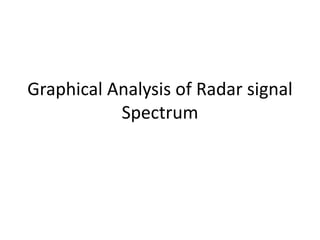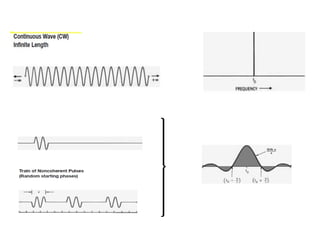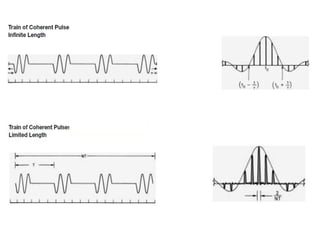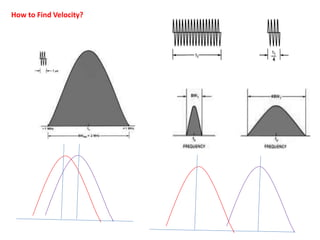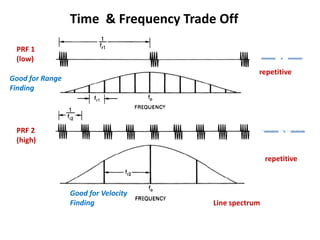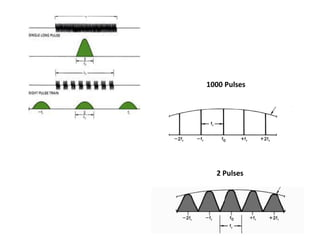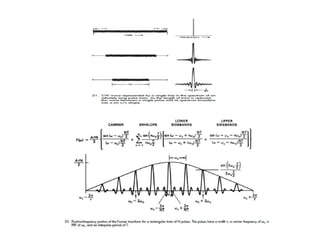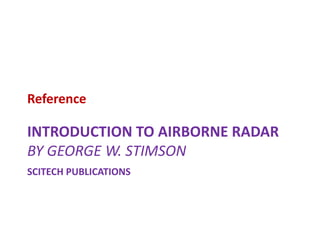1 de 36

• 2. RADAR Electro Magnetic (EM) SENSOR Uses Radio Waves Used for Detection of Objects Finding Position, vel Imaging of Objects/Gnd Tracking objects etc Complex System Multidisciplinary knowledge Hardware design follows COTS appraoch Software development comprises extensive amount of DSP concepts Acts as a Motivation for Learning and Understanding DSP Radar concepts can be used in ROBOT Radar, SONAR ,Ultrasonic ranging etc
• 3. INTRODUCTION TO RADAR RADAR = Radio Detection and Ranging • Detects targets by receipt of reflected echoes from transmitted RF pulses • Measures metric coordinates of detected targets: - Range - Doppler/velocity of targets - Angle (azimuth and/or elevation) • Special-purpose radars also measure: - Target size / shape (target imaging) - Target motion spectrum - Ground map images (SAR)
• 4. RADAR : Working Principle
• 5. Radar Measurements • Metric Coordinates of Detected Targets – Range: R = c / 2; c = speed of light, m/s = round-trip echo time, s – Range Rate: vr= fd/2 vr = target radial velocity, m/s wavelength of RF Txmit pulse, m fd= Doppler freq. shift of echo, Hz – Azimuth / Elevation – position of antenna beam at time of detection.
• 6. Radar Measurements • Measurement Resolution of Metric Coordinates – Range: R = c /(2 B) ; B = pulse bandwidth, Hz – Range Rate: vr= /(2 Tc); Tc = coherent integration tim, s – Angle: = /D ;D = antenna aperture, m ( in radians • Special-Purpose Radar Measurements – Target Motion Spectra; Modulation of Doppler shift by target motion – Synthetic Aperture Radar; Conversion of Doppler shift to angle offset
• 7. RADAR SIGNAL PROCESSING Extensive usage of DSP concepts 1. How to find range of the object/Target ? Time Domain Processing (Band Pass Sampling) 2. How to find the speed of the object/Target ? Frequency Domain Processing (DFT/FFT) 3. How to achieve desired range resolution ? Frequency Domain Processing (Fast Convolution, Windowing) 4. How to suppress Unwanted signal/Clutter ? Frequency Domain Processing (FIR Filters)
• 8. Radar On a Robot
• 10. Band Designation Frequency range (MHz) HF 3 - 30 VHF 30 - 300 UHF 300 - 1000 L 1000 - 2000 S 2000 - 4000 C 4000 - 8000 X 8000 - 12,000 Ku 12,000 - 18,000 Ka 27,000 - 40,000 mm 40 - 300GHz RADAR FREQUECY BAND Ultrasonic Ranging
• 12. RADAR : Basic Types • Co-located transmitter and Receiver with a sharing antenna • A pulse is transmitted and then the radar listens for the return • The strength of the signal is proportional to the target distance and its electrical size • The range is calculated from the time delay Single antenna Time shared by the transmitter and receiver CW Radar with independent Transmitting and Receiving antenna Continuous Wave Radars(CW) Pulsed Radars • Independent Transmitter and Receiver Bistatic Radars Monostatic Radars
• 13. RADAR WAVEFORMS Pulsed Radar Tx t ft fd ft Rx (RF) FIF fd t Rx (IF) FIF fd Rx Base band t t fd fd CW Radar Tx Rx (RF) Rx (IF) Rx Base band IF pulsed ft ft ft fd FIF fd fd Target echo train pulses
• 14. RADAR SIGNAL PROCESSING Extensive usage of DSP concepts 1. How to find range of the object/Target ? Time Domain Processing (Sampling)
• 15. R= cTR/2 Where c= velocity of light TR= Round Trip Time For , TR=1us R= ½ x 3 x 108 x 1 x 10-6 =150m. Radar TARGET DETECTION : Detection Range R
• 16. RADAR WAVEFORMS  CW : Continuous Wave  FMCW : Freq. Modulated Continuous Wave  Pulsed  Pulsed Coded T = 1/PRF = PRI Pulse Width : Pulse Repetition Interval (PRI) : T Pulse Repetition Frequency (PRF) : fr = 1/T The PRF is chosen to meet the max. unambiguous Range desired : Dist = Speed x Time 2 x Runamb max = C x T = C x 1/fr fr ≤ C/ 2.R unamb max t
• 17. Rmax proportional to [ PTG2 2 / (4 )3)Smin] PT = Transmitted power G = Gain of the antenna = Wave length = Target cross section Smin = Minimum Detection signal Rmax = [ PTAe2 /4 2 Smin] ¼ Ae = Antenna aperture RADAR RANGE EQUATION
• 18. Range measurement Radar Radar Pulse Radar jet. the to Range R n propagatio of velocity c reception and ansmission between tr delay time 2 c R • Radar measures distance by measuring time delay between the transmit and received pulse. – 1 us = 150 m – 1 ns = 15 cm RADAR PRINCIPLES: Pulsed Radar
• 19.  Radio Detection and Ranging (RADAR)  Uses Electromagnetic wave RADAR Radar R R Target Tx t TD t Distance : 2R Speed : 3x 108 m/s R = TD * 3 x 108 For 1 Km TD = 6.666 sec 1 sec delay = 150 m range
• 20. Power : Radar range Equation Pulse Width : Range Resolution Pulse Length : Unambiguous Range & Doppler Number of Pulses : Doppler and Energy Modulation inside Pulse : Range Resolution How to Find Range?
• 21. Range (A/D) Sampling Process x pulse (1/ f ) s range / time xmit xmit pulse xmit pulse target echoes pulse repetition interval (pri) N A/D Sampling Strobes r target echoes range / time N / f r s fs = A/D Sampling rate
• 22. Maximum unambiguous Range, Run=cT/2 Target return Target return T Time Time Ambiguous Range TARGET DETECTION : Maximum Range Unambiguous Range
• 23. RADAR SIGNAL PROCESSING Extensive usage of DSP concepts 1. How to find the speed of the object/Target ? Frequency Domain Processing (DFT/FFT)
• 24. Total Velocity Radial Velocity Tangential Velocity Produces Maximum Doppler Shift Zero Doppler Shift Part of the target’s velocity produces doppler shift TARGET DETECTION : Detect Velocity (Doppler Shift)
• 25. Frequency being rate of change of phase: f = 1/2 (d /dt) The echo signal suffers a change or shift in frequency called the DOPPLER FREQUENCY shift, given by : fd = 2 vr/ = 2 v. Cosθ/ DOPPLER EFFECT Any echo signal coming from a moving target suffers a change in phase with respect to time Antenna u θ vr Vr = v. cosθ Wavelength : is the distance the wave must travel to suffer a 360˚ phase change or complete one cycle : Time for 1 cycle = 1/f
• 26. SPECTRUM OF PULSED RADAR SIGNAL TIME DOMAIN : t ft ft T = 1/ fr FREQUENCY DOMAIN : fr 2/ ft - 1/ ft ft + 1/ For target echo : central line will be ft fd Each line has a spread : Spread depend on several factors but mainly dominated by inverse of antenna dwell time. fr f Expanded view Line spectrum weighted by SINC function.
• 27. 0 -PRF +PRF Doppler shift fd Echo spectral line Unambiguous doppler region Unambiguous doppler Transmit spectral line Clutter echo 0 -PRF +PRF Doppler shift fd Ambiguous doppler fa TARGET DETECTION : Doppler Ambiguity
• 28. Graphical Analysis of Radar signal Spectrum
• 31. How to Find Velocity?
• 32. PRF 1 (low) PRF 2 (high) repetitive Line spectrum repetitive Time & Frequency Trade Off Good for Range Finding Good for Velocity Finding
• 35. INTRODUCTION TO AIRBORNE RADAR BY GEORGE W. STIMSON SCITECH PUBLICATIONS Reference